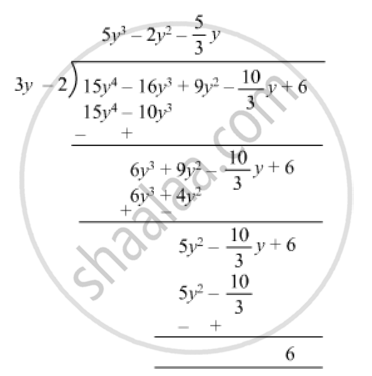Advertisement Remove all ads

# Verify the Division Algorithm I.E. Dividend = Divisor × Quotient + Remainder, in Each of the Following. Also, Write the Quotient and Remainder. Dividend Divisor 15y4 − 16y3 + 9y2 − 10 3 Y+6 3y − 2 - Mathematics

Sum

Verify the division algorithm i.e. Dividend = Divisor × Quotient + Remainder, in each of the following. Also, write the quotient and remainder.

 Dividend Divisor 15y4 − 16y3 + 9y2 −$\frac{10}{3}$ y+6 3y − 2
Advertisement Remove all ads

#### SolutionQuotient  = $5 y^3 - 2 y^2 + \frac{5}{3}y$

Remainder =  6
Divisor = 3y - 2

Divisor x  Quotient + Remainder = (3y - 2) (5y3 - 2y2 +

$\frac{5}{3}y$+ 6
$15 y^4 - 6 y^3 + 5 y^2 - 10 y^3 + 4 y^2 - \frac{10}{3}y + 6$
$15 y^4 - 16 y^3 + 9 y^2 - \frac{10}{3}y + 6$
=  Dividend
Thus,
Divisor x Quotient + Remainder = Dividend
Hence verified.
Is there an error in this question or solution?
Advertisement Remove all ads

#### APPEARS IN

RD Sharma Class 8 Maths
Chapter 8 Division of Algebraic Expressions
Exercise 8.4 | Q 21.5 | Page 12
Advertisement Remove all ads

#### Video TutorialsVIEW ALL 

Advertisement Remove all ads
Share
Notifications

View all notifications

Forgot password?
Course